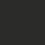# Problem 2: Symmetrical Properties of Roots

If $\alpha$ and $\beta$ are the roots of the equation $2x^2-5x-1=0$, form equations whose roots are

(a) $\frac{\alpha}{\beta}$, $\frac{\beta}{\alpha}$

(b) $\alpha^2\beta$, $\alpha\beta^2$Note by Victor Loh
4 years, 12 months ago

MarkdownAppears as
*italics* or _italics_ italics
**bold** or __bold__ bold
- bulleted- list
• bulleted
• list
1. numbered2. list
1. numbered
2. list
Note: you must add a full line of space before and after lists for them to show up correctly
paragraph 1paragraph 2

paragraph 1

paragraph 2

[example link](https://brilliant.org)example link
> This is a quote
This is a quote
    # I indented these lines
# 4 spaces, and now they show
# up as a code block.

print "hello world"
# I indented these lines
# 4 spaces, and now they show
# up as a code block.

print "hello world"
MathAppears as
Remember to wrap math in $ ... $ or $ ... $ to ensure proper formatting.
2 \times 3 $2 \times 3$
2^{34} $2^{34}$
a_{i-1} $a_{i-1}$
\frac{2}{3} $\frac{2}{3}$
\sqrt{2} $\sqrt{2}$
\sum_{i=1}^3 $\sum_{i=1}^3$
\sin \theta $\sin \theta$
\boxed{123} $\boxed{123}$

Sort by:

Before attempting to solve these, it is better to find out what $\alpha+\beta$ and $\alpha\beta$ is as we can easily see that we can try and use the Vieta's Formula to solve these.

$\alpha+\beta=2.5$

$\alpha\beta=-0.5$

Solution (a):

The equation should be in the form $x^2-(\dfrac{\alpha}{\beta}+\dfrac{\beta}{\alpha})x+1$

We can rewrite $\dfrac{\alpha}{\beta}+\dfrac{\beta}{\alpha}$ to be $\dfrac{\alpha^2+\beta^2}{\alpha\beta}$. $\alpha^2+\beta^2$ is not hard to find, as it is$(\alpha+\beta)^2-2\alpha\beta=7.25$. Then we can easily get $\dfrac{\alpha^2+\beta^2}{\alpha\beta}=\dfrac{7.25}{-0.5}=-14.5$

Therefore, $(x-\dfrac{\alpha}{\beta})(x-\dfrac{\beta}{\alpha})=\boxed{x^2+14.5x+1}$

Solution (b):

The equation should be in the form $x^2-(\alpha^2\beta+\alpha\beta^2)x+\alpha^3\beta^3$

To find $\alpha^2\beta+\alpha\beta^2$, we can factorize it to be $\alpha\beta(\alpha+\beta)$. It's lot more easier now, and we get $\alpha^2\beta+\alpha\beta^2=-1.25$

Now we need to find $\alpha^3\beta^3$, which is not so difficult as we can write it as $(\alpha\beta)^3=-0.125$

Therefore, $(x-\alpha^2\beta)(x-\alpha\beta^2)=\boxed{x^2+1.25x-0.125}$

Edited:

The equation for (a) can also be written as $2x^2+29x+2$ while the equation for (b) can be written as $8x^2+10x-1$ as it will look better.

- 4 years, 12 months ago

Nice solution! By the way, here's a challenge for you: can you generalise this to $f(x)=ax^2+bx+c$?:)

- 4 years, 12 months ago

For (a),

$f(x)=x^2-\left(\frac{\left(\frac{-b}{a}\right)^2-\frac{2c}{a}}{\frac{c}{a}}\right)x+1$

For (b),

$f(x)=x^2- \left(\frac{c}{a}\times\frac{-b}{a}\right)x+\left(\frac{c}{a}\right)^3$

- 4 years, 12 months ago

Like what? $f(x)=2x^2+29x+2$ and $f(x)=8x^2+10x-1$?

- 4 years, 12 months ago

Nope, if the coefficients of a certain quadratic equation are $a,b,c$ and its roots are $\alpha, \beta$, then construct a new equation in terms of $a,b,c$ if the roots of the new equation are

i) $\frac{\alpha}{\beta}, \frac{\beta}{\alpha}$

ii) $\alpha^2\beta, \alpha\beta^2$

- 4 years, 12 months ago

Ok, I'll try

- 4 years, 12 months ago

you could rewrite the solution for (a) to be $2x^2+29x+2$ & of (b) to be $8x^2+10x-1$. integers seem better in equations than fractions .

- 4 years, 12 months ago

oh, that's right, I will edit it

Thanks ;D

- 4 years, 12 months ago

Great :D

- 4 years, 12 months ago

Thanks

- 4 years, 12 months ago